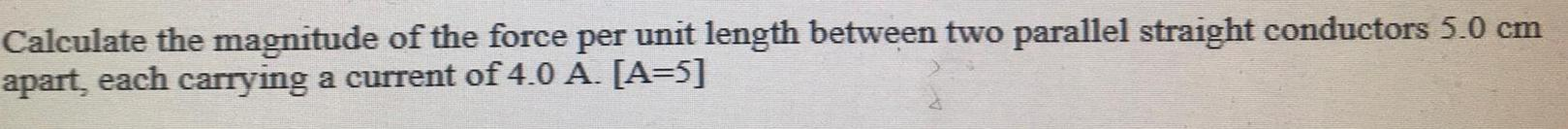Question:

# Calculate the magnitude of the force per unit length between

Last updated: 8/7/2022Calculate the magnitude of the force per unit length between two parallel straight conductors 5.0 cm apart, each carrying a current of 4.0 A. [A=5]